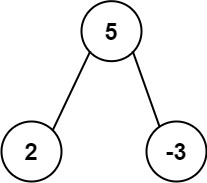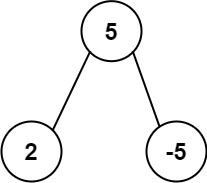# 508. Most Frequent Subtree Sum

Medium
Given the `root` of a binary tree, return the most frequent subtree sum. If there is a tie, return all the values with the highest frequency in any order.
The subtree sum of a node is defined as the sum of all the node values formed by the subtree rooted at that node (including the node itself).
Example 1:Input: root = [5,2,-3]
Output:
[2,-3,4]
Example 2:Input: root = [5,2,-5]
Output:

Constraints:
• The number of nodes in the tree is in the range `[1, 104]`.
• `-105 <= Node.val <= 105`

### 解題

Runtime: 8 ms, faster than 85.71%
Memory Usage: 6.8 MB, less than 25%
/**
* Definition for a binary tree node.
* type TreeNode struct {
* Val int
* Left *TreeNode
* Right *TreeNode
* }
*/
func findFrequentTreeSum(root *TreeNode) []int {
count := make(map[int]int)
ans := make([]int, 0)
var helper func(*TreeNode) int
helper = func(root *TreeNode) int {
if root == nil { return 0 }
sum := helper(root.Left) + helper(root.Right) + root.Val
if len(ans) == 0 || count[sum]+1 == count[ans]{
ans = append(ans, sum)
} else if count[sum]+1 > count[ans] {
ans = []int{ sum }
}
count[sum]++
return sum
}
helper(root)
return ans
}